This is a continuation from the previous tutorial - radiative recombination in semiconductors.

As mentioned in the radiative recombination in semiconductors tutorial, electrons and holes in a semiconductor can also recombine nonradiatively.

Nonradiative mechanisms include recombination at defects, surface recombination, and Auger recombination, among others. For long-wavelength semiconductor lasers, however, the Auger process is generally the predominant nonradiative mechanism, and most of this tutorial is devoted to its study. The surface- and defect-recombination processes are discussed briefly too.

The Auger recombination process involves four particle states (three electrons and one hole, two electrons and two holes, and so forth). In this process, the energy released during the electron-hole recombination is transferred to another electron (or hole), which gets excited to a high energy state in the band. This electron or hole then relaxes back to achieve thermal equilibrium by losing its energy to lattice vibrations or phonons.

The Auger recombination rate $$R_\text{a}$$ may be approximately written as

$\tag{3-3-1}R_\text{a}=Cn^3$

where $$n$$ is the injected carrier density in an undoped semiconductor.

It is useful to define the carrier lifetime $$\tau_\text{A}$$ for the Auger process as

$\tag{3-3-2}\tau_\text{A}=n/R_\text{a}=(Cn^2)^{-1}$

The quantity $$C$$ is called the Auger coefficient.

The inverse-square dependence of carrier lifetime on carrier density is used to identify the Auger effect experimentally. Departures from the simple inverse-square relation are discussed later in the section.

It is generally accepted that Auger recombination can be a major nonradiative mechanism in narrow-gap semiconductors. The observed strong temperature dependence of threshold current in long-wavelength semiconductor lasers has focused attention on the Auger effect.

There are several different types of Auger recombination processes. The three major types are

1. Band-to-band processes
2. Phonon-assisted Auger processes
3. Trap-assisted processes

## Band-to-Band Auger Processes

The band-to-band Auger processes in a direct-gap semiconductor are shown in Figure 3-13.

The three processes are labeled CCCH, CHHS, and CHHL where C stands for the conduction band and H, L, and S stand for heavy-hole, light-hole, and split-off valence bands, respectively.

The CCCH process involves three electrons and one hole and is dominant in n-type material.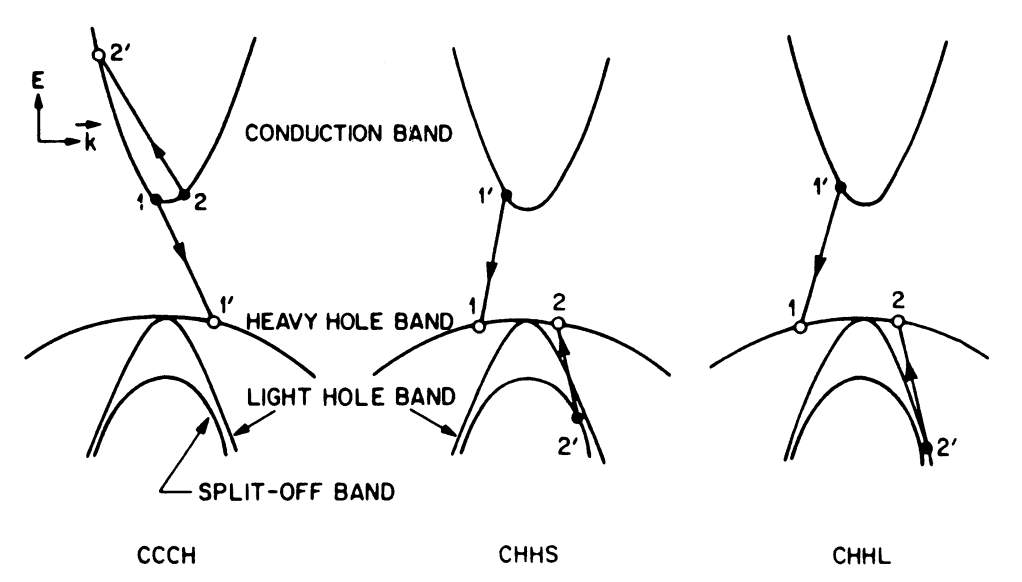Figure 3-13.  Three different band-to-band Auger processes shown schematically. Electrons are represented by closed circles and holes by open circles.

The basis of the Auger effect is the Coulomb interaction between the two electrons $$1$$ and $$2$$ in the conduction band. In the CCCH mechanism, electron $$1$$ recombines with hole $$1'$$ (i.e., electron $$1$$ makes a transition to empty state $$1'$$) and the excess energy is transferred to electron $$2$$, which is excited to state $$2'$$. Electron $$2'$$ loses its energy to optical phonons when it relaxes back to thermal equilibrium.

The CHHS process involves one electron, two heavy holes, and a split-off-band hole. CHHL is similar to CHHS except that it involves a light hole. CHHS and CHHL are dominant in p-type semiconductors.

Under the high-injection conditions commonly present in semiconductor lasers, all three mechanisms must be considered.

Band-to-band Auger processes are characterized by a strong temperature dependence and band-gap dependence, i.e., the Auger rate decreases rapidly either for low temperatures or for high band-gap materials.

These dependencies arise from the laws of energy and momentum conservation that the four free particle states involved ($$1$$, $$2$$, $$1'$$, $$2'$$ in Figure 3-13) must satisfy.

This may be seen in the following way for CCCH.

The momentum and energy conservation laws give rise to a threshold energy $$E_\text{T}$$ for each process. For CCCH, if we assume $$E_1\approx{E}_2=0$$, only holes with energies greater than $$\sim(E_\text{T}-E_\text{g})=\delta{E}_\text{g}$$ can participate ($$\delta$$ is a constant that depends on effective masses).

The number of such holes varies approximately as $$\exp(-\delta{E}_\text{g}/k_\text{B}T)$$ for nondegenerate semiconductors, and thus the Auger rate varies as $$\exp(-\delta{E}_\text{g}/k_\text{B}T)$$ in the nondegenerate case.

The strong temperature dependence does not appear if the individual particle states are not states of definite momentum, such as trap states, or if conservation of momentum is satisfied through phonon assistance. These processes are discussed later in this tutorial.

We now calculate the recombination rates of the various band-to-band Auger processes.

CCCH Auger Process

Beattie and Landsberg derived an expression for the CCCH Auger process using Bloch functions for particle states. Using Fermi's golden rule, the expression for the Auger rate is

\tag{3-3-3}\begin{align}R_\text{a}=&\frac{2\pi}{\hbar}\left(\frac{1}{(2\pi)^3}\right)^4\displaystyle\iiiint|M_\text{if}|^2P(1,1',2,2')\\&\times\delta(\mathbf{k}_1+\mathbf{k}_2-\mathbf{k}_1'-\mathbf{k}_2')\delta(E_\text{i}-E_\text{f})\text{d}^3\mathbf{k}_1\text{d}^3\mathbf{k}_2\text{d}^3\mathbf{k}_1'\text{d}^3\mathbf{k}_2'\end{align}

where $$R_\text{a}$$ denotes the Auger rate per unit volume. $$P(1,1',2,2')$$ denotes the net probability for the CCCH process.

The momentum conservation (represented by the first $$\delta$$ function) arises from the representation of the particle states as the product of a plane wave and a Bloch function.

$$M_\text{if}$$ is the matrix element of the Coulomb interaction potential $$V$$ between two electrons, i.e.,

$\tag{3-3-4}V(\mathbf{r}_1-\mathbf{r}_2)=\frac{q^2}{\epsilon|\mathbf{r}_1-\mathbf{r}_2|}\exp[-\lambda|\mathbf{r}_1-\mathbf{r}_2|]$

where $$\lambda$$ is the inverse of the screening length, $$\epsilon$$ is the dielectric constant, and $$\mathbf{r}_1$$ and $$\mathbf{r}_2$$ are the positions of the interacting electrons.

The matrix element after summing over the singlet and triplet state of electrons $$1$$ and $$2$$ takes the following form:

\tag{3-3-5}\begin{align}M_\text{if}=&\displaystyle\iint[\phi_1^*(\mathbf{r}_1)\phi_2^*(\mathbf{r}_2)\Delta_{21}-\phi_2^*(\mathbf{r}_1)\phi_1^*(\mathbf{r}_2)\Delta_{12}]\\&\times{V}(\mathbf{r}_1-\mathbf{r}_2)\phi_1'(\mathbf{r}_1)\phi_2'(\mathbf{r}_2)\text{d}^3\mathbf{r}_1\text{d}^3\mathbf{r}_2\end{align}

where $$\phi_1(\mathbf{r}_1)$$ and $$\phi_2(\mathbf{r}_2)$$ denote the initial state of electrons $$1$$ and $$2$$ while $$\phi_1'(\mathbf{r}_1)$$, $$\phi_2'(\mathbf{r}_2)$$ denote the corresponding final states.

The quantities $$\Delta_{21}=\Delta_{12}=1$$ when electrons $$1$$ and $$2$$ have like spins, $$\Delta_{21}=1$$, $$\Delta_{12}=0$$ when they have opposite spins preserved by the transition, and $$\Delta_{12}=1$$, $$\Delta_{21}=0$$ when they have opposite spins both changed by the transition.

The wave functions $$\phi_1(\mathbf{r}_1)$$ can be written as

$\tag{3-3-6}\phi_1(\mathbf{r}_1)=\psi_1(\mathbf{k}_1,\mathbf{r}_1)\exp(\text{i}\mathbf{k}_1\cdot\mathbf{r}_1)$

where $$\psi_1(\mathbf{k}_1,\mathbf{r}_1)$$ denotes the localized part of the Block function and $$\exp(\text{i}\mathbf{k}_1\cdot\mathbf{r}_1)$$ is the plane-wave part.

Consider one term in the matrix element and carry out the integration after using Equations (3-3-4) and (3-3-6). The result is

\tag{3-3-7}\begin{align}\displaystyle\iint&\phi_1^*(\mathbf{r}_1)\phi_2^*(\mathbf{r}_2)V(\mathbf{r}_1-\mathbf{r}_2)\phi_1'(\mathbf{r}_1)\phi_2'(\mathbf{r}_2)\text{d}^3\mathbf{r}_1\text{d}^3\mathbf{r}_2\\&=\delta(\mathbf{k}_1+\mathbf{k}_2-\mathbf{k}'_1-\mathbf{k}'_2)\frac{4\pi{q^2}}{\epsilon(\lambda^2+g^2)}F(1,1')F(2,2')\end{align}

where $$g=|\mathbf{k}_1-\mathbf{k}'_1|$$ and $$F(1,1')$$ and $$F(2,2')$$ are the overlap integrals defined using

\begin{align}F(1,1')=\displaystyle\int\psi_1^*(\mathbf{r}_1)\psi'_1(\mathbf{r}_1)\text{d}^3\mathbf{r}_1\\F(2,2')=\displaystyle\int\psi_2^*(\mathbf{r}_2)\psi'_2(\mathbf{r}_2)\text{d}^3\mathbf{r}_2\\\end{align}

In deriving the above, we have used the relation $$\mathbf{r}_{12}=\mathbf{r}_1-\mathbf{r}_2$$ and the integral

$\tag{3-3-8}\displaystyle\int\frac{\text{d}^3\mathbf{r}_{12}}{r_{12}}\exp(-\lambda{r_{12}}+\text{i}\mathbf{g}\cdot\mathbf{r}_{12})=\frac{4\pi}{\lambda^2+g^2}$

Using a similar procedure for the other terms in Equation (3-3-5), the matrix element $$|M_\text{if}|^2$$ is given by

\tag{3-3-9}\begin{align}|M_\text{if}|^2=\left(\frac{4\pi{q^2}}{\epsilon}\right)^2\left[\left|\frac{F(1,1')F(2,2')}{\lambda^2+g^2}\right|^2+\left|\frac{F(1,2')F(2,1')}{\lambda^2+h^2}\right|^2\right.\\\left.+\left|\frac{F(1,1')F(2,2')}{\lambda^2+g^2}-\frac{F(1,2')F(2,1')}{\lambda^2+h^2}\right|^2\right]\end{align}

where $$g^2=|\mathbf{k}_1-\mathbf{k}'_1|^2=|\mathbf{k}'_2-\mathbf{k}_2|^2$$ and $$h^2=|\mathbf{k}_1-\mathbf{k}'_2|^2=|\mathbf{k}'_1-\mathbf{k}_2|^2$$.

$$F(i,j)$$'s denote the overlap integral of the Bloch functions. These overlap integrals have been evaluated using the Kane model for the band structure. The result is that

$\tag{3-3-10a}|F(i,j)|^2\approx1$

when $$i$$ and $$j$$ are in the same band, and

$\tag{3-3-10b}|F(i,j)|^2\approx\frac{\alpha_{ij}}{E_\text{g}}|\mathbf{k}_i-\mathbf{k}_j|^2$

when $$i$$ and $$j$$ are in different bands. Here $$\alpha_{ij}$$ is a contant.

Calculations of the overlap integrals using a more detailed band structure have been carried out. Since the matrix element given by Equation (3-3-9) is fairly complicated, some approximate form is generally employed.

An approximation to the matrix element $$|M_\text{if}|^2$$ obtained using Equation (3-3-10) is $$(0.5|M'_\text{if}|^2\le|M_\text{if}|^2\le1.5|M'_\text{if}|^2)$$:

$\tag{3-3-11}|M_\text{if}|^2\approx|M'_\text{if}|^2=4\left(\frac{4\pi{q^2}}{\epsilon}\right)^2\frac{\alpha_\text{CH}}{E_\text{g}}\frac{k_2'^2}{|k_2'^2+\lambda^2|^2}=4|\overline{M}_\text{if}|^2$

Here $$\alpha_\text{CH}=\alpha'_{11}$$, and we have used the approximation $$k_2'^2\gg{k}_1^2,k_2^2$$ so that $$g^2=h^2=k_2'^2$$.

Equation (3-3-11) defines the matrix element $$|M_\text{if}|^2$$, and the expression (3-3-3) for the Auger rate can be written as

\tag{3-3-12}\begin{align}R_\text{a}=&\left(\frac{8\pi}{\hbar}\right)\left(\frac{1}{8\pi^3}\right)^4\displaystyle\iiiint|\overline{M}_\text{if}|^2P(1,1',2,2')\\&\times\delta(\mathbf{k}_1+\mathbf{k}_2-\mathbf{k}'_1-\mathbf{k}'_2)\delta(E_\text{i}-E_\text{f})\text{d}^3\mathbf{k}_1\text{d}^3\mathbf{k}_2\text{d}^3\mathbf{k}_1'\text{d}^3\mathbf{k}_2'\end{align}

The quantity $$P(1,1',2,2')$$ in Equation (3-3-12) is the difference between the probabilities of the Auger recombination process of an electron-hole pair shown in Figure 3-13 and the inverse process of creating a pair.

For the CCCH process to occur, there must be electrons in states $$|1\rangle$$, $$|2\rangle$$, a hole in state $$|1'\rangle$$, and no electrons in state $$|2'\rangle$$. The appropriate occupation probabilities are given by the Fermi factors (3-2-2) and (3-2-3) [refer to the radiative recombination in semiconductors tutorial]. Using them, we obtain

\tag{3-3-13}\begin{align}P(1,1',2,2')=&f_\text{c}(1)f_\text{c}(2)f_\text{v}(1')[1-f_\text{c}(2')]\\&-[1-f_\text{c}(1)][1-f_\text{c}(2)][1-f_\text{v}(1')]f_\text{c}(2')\end{align}

where $$f_\text{c}(i)$$ stands for $$f_\text{c}(E_\text{i})$$, $$E_\text{i}$$ being the energy of $$i$$th particle.

The second term in Equation (3-3-13) is almost negligible since $$f_\text{c}(2')\approx0$$ in most cases of practical interest where $$E_\text{g}\gg{k}_\text{B}T$$.

To a good degree of approximation, we can therefore use

$\tag{3-3-14}P(1,1',2,2')\approx{f_\text{c}(1)}f_\text{c}(2)f_\text{v}(1')[1-f_\text{c}(2')]$

The energies of the four particles involved in the Auger process satisfy the energy conservation relation

$\tag{3-3-15}E_1+E_2=E_2'-E_\text{g}-E_1'$

Note that the hole energies are measured positive downward from the top of the valence band.

For the nondegenerate case, the probability factor $$P(1,1',2,2')$$ can be simplified using Equations (3-2-30) and (3-2-33) [refer to the radiative recombination in semiconductors tutorial]. The result is

$\tag{3-3-16}P(1,1',2,2')=\frac{n^2p}{N_\text{c}^2N_\text{v}}\exp\left(\frac{-E_2'+E_\text{g}}{k_\text{B}T}\right)$

where we have used Equation (3-3-15) and assumed that $$f_\text{c}(2')\ll1$$.

The total Auger recombination rate is now given by

\tag{3-3-17}\begin{align}R_\text{a}=&\frac{8\pi}{\hbar}\left(\frac{1}{8\pi^3}\right)^4\xi_1\displaystyle\iiiint\exp\left(\frac{-E_2'}{k_\text{B}T}\right)|\overline{M}_\text{if}(k_2')|^2\\&\times\delta(\mathbf{k}_1+\mathbf{k}_2-\mathbf{k}_1'-\mathbf{k}_2')\delta(E_\text{i}-E_\text{f})\text{d}^3\mathbf{k}_1\text{d}^3\mathbf{k}_2\text{d}^3\mathbf{k}_1'\text{d}^3\mathbf{k}_2'\end{align}

where we have assumed that $$\overline{M}_\text{if}$$ depends only on $$k_2'$$ as in Equation (3-3-11) and $$\xi_1$$ is given by

$\tag{3-3-18}\xi_1=\frac{n^2p}{N_\text{c}^2N_\text{v}}\exp\left(\frac{E_\text{g}}{k_\text{B}T}\right)$

The integrals can be considerably simplified if we define the new variables

$\mathbf{h}=\mathbf{k}_1+\mathbf{k}_2\qquad\mathbf{j}=\mathbf{k}_1-\mathbf{k}_2$

Integrating over them, we obtain

$\tag{3-3-19}R_\text{a}=\frac{m_\text{c0}\xi_1}{64\pi^7\hbar^3}\displaystyle\iint\text{d}^3\mathbf{k}_1'\text{d}^3\mathbf{k}_2'|j_0||\overline{M}_\text{if}|^2\exp\left(\frac{-E_2'}{k_\text{B}T}\right)$

where

\begin{align}j_0^2&=2\left[\mu_\text{c}k_2'^2-\mu{k}_1'^2-\frac{2m_\text{c0}E_\text{g}}{\hbar^2}\right]-|\mathbf{k}_1'+\mathbf{k}_2'|^2\\\mu_\text{c}&=\frac{m_\text{c0}}{m_\text{c}}\qquad\mu=\frac{m_\text{c0}}{m_\text{v}}\end{align}

Here $$m_\text{c0}$$ and $$m_\text{c}$$ are the conduction-band effective masses at the band edge and at an energy $$E_2'$$, respectively.

Defining the new variables

$\tag{3-3-20}\mathbf{Z}_1=\mathbf{k}_1'+\frac{\mathbf{k}_2'}{1+2\mu}\qquad\mathbf{Z}_2=\mathbf{k}_2'$

we get the following result:

$\tag{3-3-21}R_\text{a}=\frac{m_\text{c0}\xi_1}{4\pi^5\hbar^3}I$

where

$\tag{3-3-22}I=\displaystyle\iint\text{d}Z_1\text{d}Z_2Z_1^2Z_2^2|J_0||\overline{M}_\text{if}|^2\exp\left(\frac{-E_2'}{k_\text{B}T}\right)$

and

$\tag{3-3-23}J_0^2=2\alpha_\text{c}Z_2^2-Z_1^2(1+2\mu)-\frac{4m_\text{c0}E_\text{g}}{\hbar^2}$

where

$\alpha_\text{c}=\mu_\text{c}-\frac{\mu}{1+2\mu}$

Before simplifying the integral $$I$$ further, we note the following.

Since $$I$$ should be real, $$J_0^2$$ must be a positive quantity. Since $$E_2'=\hbar^2Z_2^2/2m_\text{c}$$, Equation (3-3-23) for $$J_0^2$$ shows that there is a minimum value of $$E_2'$$ for the Auger process to take place. A lower bound on this minimum value is obtained using

$2\alpha_\text{c}Z_2^2\gt\frac{4m_\text{c0}E_\text{g}}{\hbar^2}\qquad\text{or}\qquad{E}_2'\gt\frac{\mu_\text{c}E_\text{g}}{\alpha_\text{c}}$

Thus the threshold energy, or the minimum energy for the electron $$2'$$, is given by

$\tag{3-3-24}E_\text{T}=\frac{\mu_\text{c}E_\text{g}}{\alpha_\text{c}}=\frac{2m_\text{c0}+m_\text{v}}{2m_\text{c0}+m_\text{v}-m_\text{c}}E_\text{g}$

Most of the contribution to the integral $$I$$ arises from energies $$E_2'\approx{E}_\text{T}$$ because of the exponential term $$\exp(-E_2'/k_\text{B}T)$$ in Equation (3-3-22). Hence we can approximate $$|\overline{M}_\text{if}(k_2')|^2$$ by its value $$|M_\text{a}|^2$$ at energy $$E_\text{T}$$, i.e.,

$\tag{3-3-25}|\overline{M}_\text{if}(k_2')|^2\approx|M_\text{a}|^2$

Integration over $$Z_1$$ can be readily performed and Equation (3-3-22) becomes

$I=\frac{\pi\alpha_\text{c}^2|M_\text{a}|^2}{(1+2\mu)^{3/2}}\displaystyle\int\limits_{Z_\text{2t}}^\infty\text{d}Z_2(Z_2^2-Z_\text{2t}^2)^2Z_2^2\exp\left(\frac{-E_2'}{k_\text{B}T}\right)$

where $$Z_\text{2t}=(2m_\text{c}/\hbar^2)E_\text{T}$$.

Defining $$y=Z_2-Z_\text{2t}$$ as the new integration variable, we obtain

$\tag{3-3-26a}I=\frac{\pi\alpha_\text{c}^2|M_\text{a}|^2}{(1+2\mu)^{3/2}}\exp\left(\frac{-E_\text{T}}{k_\text{B}T}\right)I_0$

where

$\tag{3-3-26b}I_0=\displaystyle\int\limits_0^\infty\text{d}yy^2(Z_\text{2t}+y)^2(2Z_\text{2t}+y)^2\exp\left(-\frac{\hbar^2y}{2m_\text{c}k_\text{B}T}(2Z_\text{2t}+y)\right)$

and can be analytically evaluated.

Using Equations (3-3-21) and (3-3-26), the expression for the Auger rate in the nondegenerate approximation becomes

$\tag{3-3-27}R_\text{a}=\frac{m_\text{c0}\alpha_\text{c}^2|M_\text{a}|^2}{4\pi^4\hbar^3(1+2\mu)^{3/2}}\frac{n^2pI_0}{N_\text{c}^2N_\text{v}}\exp\left(\frac{-\Delta{E}}{k_\text{B}T}\right)$

where $$\Delta{E}=E_\text{T}-E_\text{g}$$.

The main points to note are that the Auger rate varies at $$n^2p$$ and is temperature-dependent through the exponential term. In particular, the Auger rate increases with increasing temperature in the nondegenerate approximation. For the degenerate case, the Auger-rate calculations can be done using the same procedure as above except that the integral $$I$$ in Equation (3-3-22) is modified.

CHHS Auger Process

The Auger recombination rate for CHHS, which involves two heavy holes, one electron and one split-off-band hole, can be calculated using the same procedure as for CCCH.

The expression for the Auger rate is given by Equation (3-3-12) with the matrix element (obtained using the Kane model):

$\tag{3-3-28}|\overline{M}_\text{if}|^2\approx\left(\frac{4\pi{q^2}}{\epsilon}\right)^2\frac{\alpha_\text{CH}}{E_\text{g}}\frac{\alpha_\text{SH}}{E_\text{g}}\frac{k_2'^4}{(k_2'^2+\lambda^2)^2}$

where we have made the approximation that $$k_2'\gg{k}_1,k_2$$.

In the nondegenerate approximation, the probability factor $$P(1,1',2,2')$$ can be simplified using Equations (3-2-30) and (3-2-33) [refer to the radiative recombination in semiconductors tutorial] to obtain

$\tag{3-3-29}P(1,1',2,2')=\frac{np^2}{N_\text{c}N_\text{v}^2}\exp\left(\frac{-E_2'+E_\text{g}}{k_\text{B}T}\right)$

The total Auger recombination rate $$R_\text{a}$$ using Equations (3-3-12) and (3-3-29) is given by

\tag{3-3-30}\begin{align}R_\text{a}=&\frac{8\pi}{\hbar}\left(\frac{1}{8\pi^3}\right)^4\xi_\text{s}\displaystyle\iiiint\exp\left(\frac{-E_2'}{k_\text{B}T}\right)|\overline{M}_\text{if}(k_2')|^2\\&\times\delta(\mathbf{k}_1+\mathbf{k}_2-\mathbf{k}_1'-\mathbf{k}_2')\delta(E_\text{i}-E_\text{f})\text{d}^3\mathbf{k}_1\text{d}^3\mathbf{k}_2\text{d}^3\mathbf{k}_1'\text{d}^3\mathbf{k}_2'\end{align}

where $$\xi_\text{s}$$ is defined by

$\tag{3-3-31}\xi_\text{s}=\frac{np^2}{N_\text{c}N_\text{v}^2}\exp\left(\frac{E_\text{g}}{k_\text{B}T}\right)$

We have made the approximation that the matrix element $$|\overline{M}_\text{if}|^2$$ depends on $$k_2'$$ in accordance with Equation (3-3-28).

The integrals in Equation (3-3-30) can be simplified using the same procedure as for the CCCH process. We define the variables $$\mathbf{h}$$ and $$\mathbf{j}$$ as before, such that $$\mathbf{h}=\mathbf{k}_1+\mathbf{k}_2$$ and $$\mathbf{j}=\mathbf{k}_1-\mathbf{k}_2$$, and integrate over them. If we further define new variables

$\tag{3-3-32}\mathbf{z}_1=\mathbf{k}_1'+\frac{\mathbf{k}_2'}{2/\mu+1}\qquad\mathbf{z}_2=\mathbf{k}_2'$

we obtain

$\tag{3-3-33}R_\text{a}=\frac{m_\text{c0}\xi_\text{s}}{4\pi^5\hbar^3\mu}I$

where

$\tag{3-3-34}I=\displaystyle\iint\text{d}z_2\text{d}z_1z_1^2z_2^2|\overline{M}_\text{if}|^2|J_0|\exp\left(\frac{-E_2'}{k_\text{B}T}\right)$

and

$\tag{3-3-35}J_0^2=\frac{2}{\mu}\alpha_\text{s}z_2^2-\frac{2}{\mu}(1+\mu/2)z_1^2-\frac{4m_\text{c0}}{\hbar^2\mu}(E_\text{g}-\Delta)$

$\tag{3-3-36}\alpha_\text{s}=\mu_\text{s}-1+\left(\frac{1}{1+\mu/2}\right)\qquad\mu_\text{s}=\frac{m_\text{c0}}{m_\text{s}}\qquad\mu=\frac{m_\text{c0}}{m_\text{v}}$

For $$J_0^2$$ to be positive, there is a minimum value of $$z_2$$ (assuming $$E_\text{g}\gt\Delta$$) for the Auger process to take place. This minimum value is obtained using

$\alpha_\text{s}z_2^2\gt2m_\text{c0}(E_\text{g}-\Delta)/\hbar^2$

Since $$E_2'=\hbar^2z_2^2/2m_\text{s}$$, the above inequality implies that $$E_2'$$ must be greater than the threshold energy $$E_\text{T}$$ for CHHS given by

$\tag{3-3-37}E_\text{T}=\frac{m_\text{c0}(E_\text{g}-\Delta)}{\alpha_\text{s}m_\text{s}}=\frac{2m_\text{v}+m_\text{c0}}{2m_\text{v}+m_\text{c0}-m_\text{s}}(E_\text{g}-\Delta)$

Making the same approximation as for the CCCH process, viz. replace $$|\overline{M}_\text{if}|^2$$ by its value $$|M_\text{s}|^2$$ at $$E_2'=E_\text{T}$$, the integral $$I$$ may be simplified to

$\tag{3-3-38}I=\frac{\pi\alpha_\text{s}^2|M_\text{s}|^2}{(1+\mu/2)^{3/2}}\left(\frac{2}{\mu}\right)^{1/2}\exp\left(\frac{-E_\text{T}}{k_\text{B}T}\right)I_0$

where $$I_0$$ is given by Equation (3-3-26b) except that $$m_\text{c}$$ is replaced by $$m_\text{s}$$.

Using Equations (3-3-31), (3-3-33), and (3-3-38), the expression for the CHHS Auger rate in the nondegenerate approximation is given by

$\tag{3-3-39}R_\text{a}=\frac{m_\text{c0}\alpha_\text{s}^2|M_\text{s}|^2}{4\pi^4\hbar^3\mu(1+\mu/2)^{3/2}}\left(\frac{2}{\mu}\right)^{1/2}\frac{np^2I_0}{N_\text{c}^2N_\text{v}}\exp\left(-\frac{\Delta{E}}{k_\text{B}T}\right)$

where

$\tag{3-3-40}\Delta{E}=E_\text{T}-E_\text{g}$

The temperature dependence and the band-gap dependence of the CHHS Auger process arises from the exponential term in Equation (3-3-39).

For the case where $$E_\text{g}\lt\Delta$$, there is no threshold energy for CHHS. The expression for the CHHS Auger rate under degeneracy has also been derived.

CHHL Auger Process

The expression of the3 Auger rate for CHHL is similar to that for CHHS except that the split-off-band mass $$m_\text{s}$$ is replaced by the light-hole mass $$m_\text{l}$$ and $$E_\text{g}-\Delta$$ is replaced by $$E_\text{g}$$ in Equation (3-3-37).

The threshold energy for CHHL is given by

$\tag{3-3-44}E_\text{T}=\frac{2m_\text{v}+m_\text{c0}}{2m_\text{v}+m_\text{c0}-m_\text{l}}E_\text{g}$

The quantities $$m_\text{c}$$, $$m_\text{s}$$, and $$m_\text{l}$$ that appear in Equations (3-3-24), (3-3-37), and (3-3-41) are the effective masses in the conduction, split-off, and light-hole bands at the threshold energies of CCCH, CHHS, and CHHL, respectively.

Since the band structure away from the band edge is not well known experimentally, simplified theoretical models must be used to calculate these effective masses. As a result, the accuracy of the calculated Auger rate is limited by the accuracy of the band-structure model used.

As stated previously, the Auger effect is mainly responsible for the observed high temperature dependence of the threshold current in long-wavelength semiconductor lasers, in particular, lasers fabricated using the InGaAsP material system and emitting near 1.3 μm and 1.55 μm.

We now present the results of calculated Auger lifetimes in InGaAsP latice-matched to InP under low injection.

The Auger lifetime of injected carriers is obtained using Equation (3-3-2) after the Auger rate $$R_\text{a}$$ has been calculated for a given carrier density $$n$$.

For n-type semiconductors, the CCCH process is important. Figure 3-14 shows the calculated Auger lifetime for n-type InGaAsP (curve labeled CCCH), with a majority carrier concentration for $$n_0=10^{18}\text{ cm}^{-3}$$. This calculation is for low injection ($$n=10^{15}\text{ cm}^{-3}$$).

For p-type semiconductors, both CHHS and CHHL may be important. Figure 3-14 shows the calculated result for both processes at low injection ($$n=10^{15}\text{ cm}^{-3}$$) for p-type InGaAsP with a majority carrier concentration of $$p_0=10^{18}\text{ cm}^{-3}$$.

In all cases the Auger lifetimes decreases (or the Auger recombination rate increases) rapidly with decreasing band gap.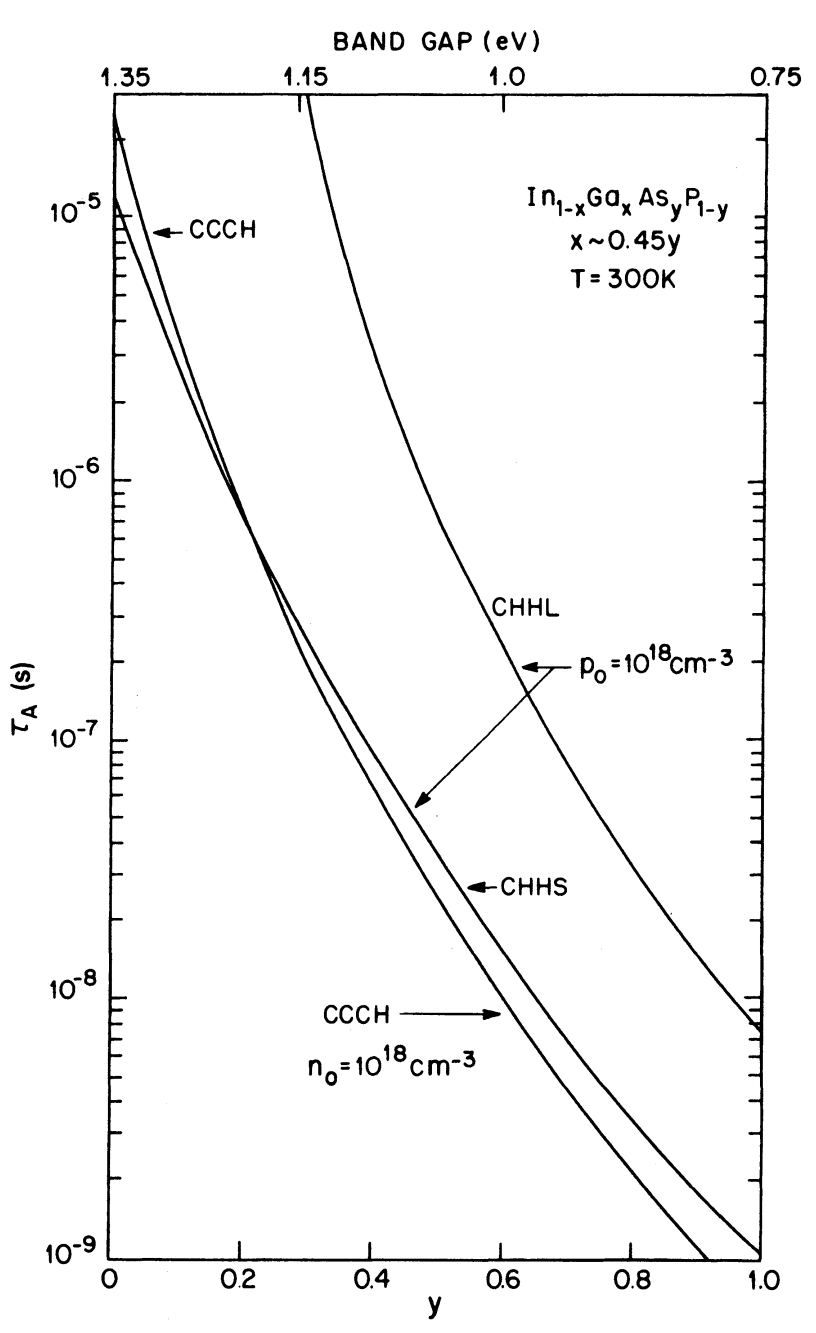Figure 3-14.  Calculated Auger lifetime $$\tau_\text{A}$$ for n-type and p-type InGaAsP at low injection as a function of band gap (top scale) or mole fraction (bottom scale) with a majority carrier concentration of $$10^{18}\text{ cm}^{-3}$$. For the n-type material CCCH dominates; for p-type material, both CHHS and CHHL are considered.

Figure 3-15 shows the temperature dependence of the Auger processes under low injection. The three curves correspond to the three Auger processes. The band gap is chosen to correspond to $$\lambda=1.3$$ μm. The Auger lifetimes decrease (or the Auger rates increase) rapidly with increasing temperature.

The temperature dependence and the band-gap dependence essentially arise from the conservation of momentum and energy that the four particle states must satisfy.

Figures 3-14 and 3-15 show qualitatively that the Auger effect is expected to play an important role in the performance of long-wavelength devices, particularly semiconductor lasers (such as InGaAsP lasers) that operate at higher carrier densities.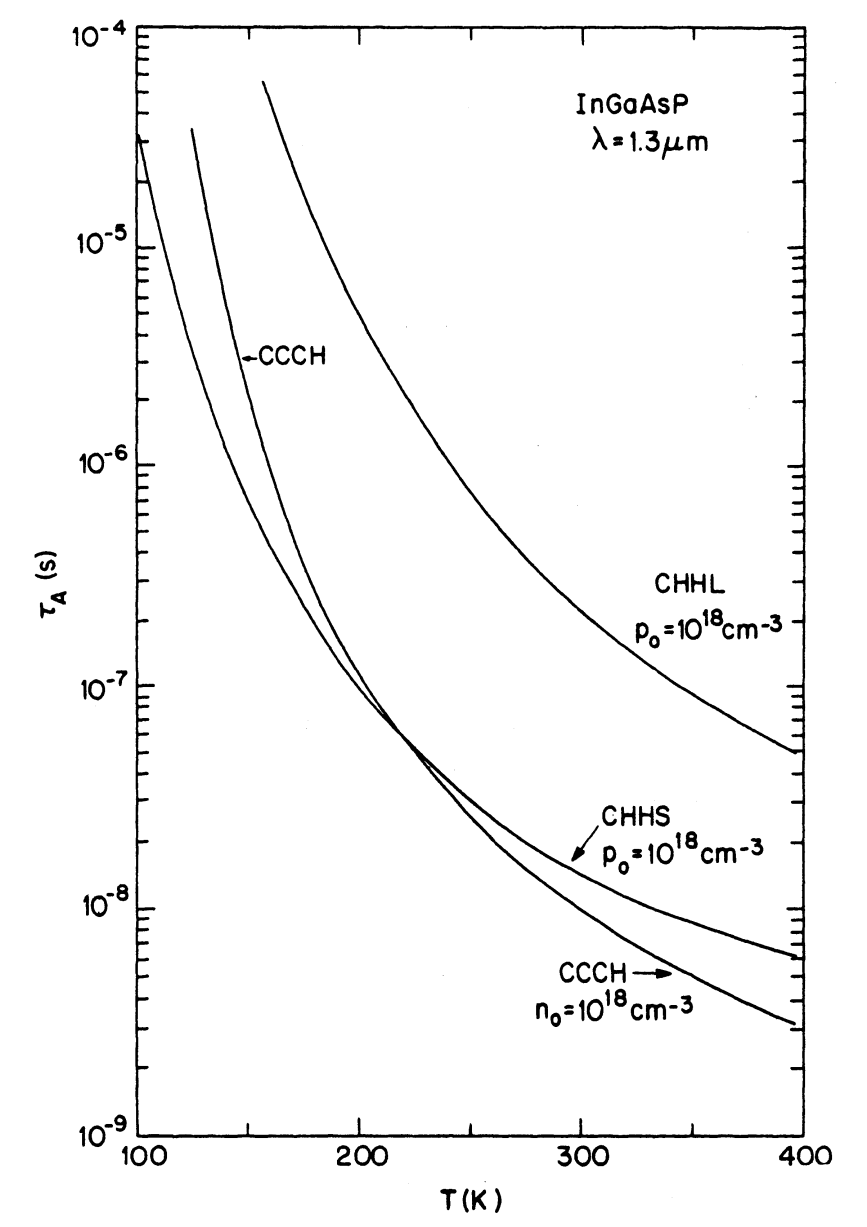Figure 3-15.  Calculated Auger lifetime for n-type and p-type InGaAsP as a function of temperature. The majority carrier concentration is $$10^{18}\text{ cm}^{-3}$$.

The screening of the Coulomb interactions and the use of the Fermi-Dirac statistics can alter the Auger rate at high injection from that expected by simple extrapolation at low injection.

Figure 3-16 shows the calculated Auger coefficient for the CHHS Auger processes as a function of injected carrier concentration for undoped In1-xGaxAsyP1-y lattice-matched to InP ($$x\approx0.45y$$).

Different curves correspond to difference values of the mole fraction $$y$$. The Auger coefficient $$C$$ is obtained using the relation $$R_\text{a}=Cn^3$$.

In the gain and stimulated emission of semiconductor lasers tutorial we used $$C$$ as a phenomenological parameter [see Equation (2-4-9)]. Figure 3-16 can be used to estimate its numerical value.

If we use a typical value for $$n$$ of $$2\times10^{18}\text{ cm}^{-3}$$ for the injected carrier density in InGaAsP lasers, we find that $$C\approx2\times10^{-28}\text{ cm}^6/\text{s}$$ at $$\lambda=1.3$$ μm ($$y\approx0.6$$) and $$C\approx5\times10^{-28}\text{ cm}^6/\text{s}$$ at $$\lambda=1.55$$ μm ($$y\approx0.95$$).

In the next tutorial we discuss the values of $$C$$ deduced experimentally. It should be stressed that because of various approximations and uncertainties in the band-structure parameters, the numerical calculations provide only an order-of-magnitude estimate of the Auger coefficient.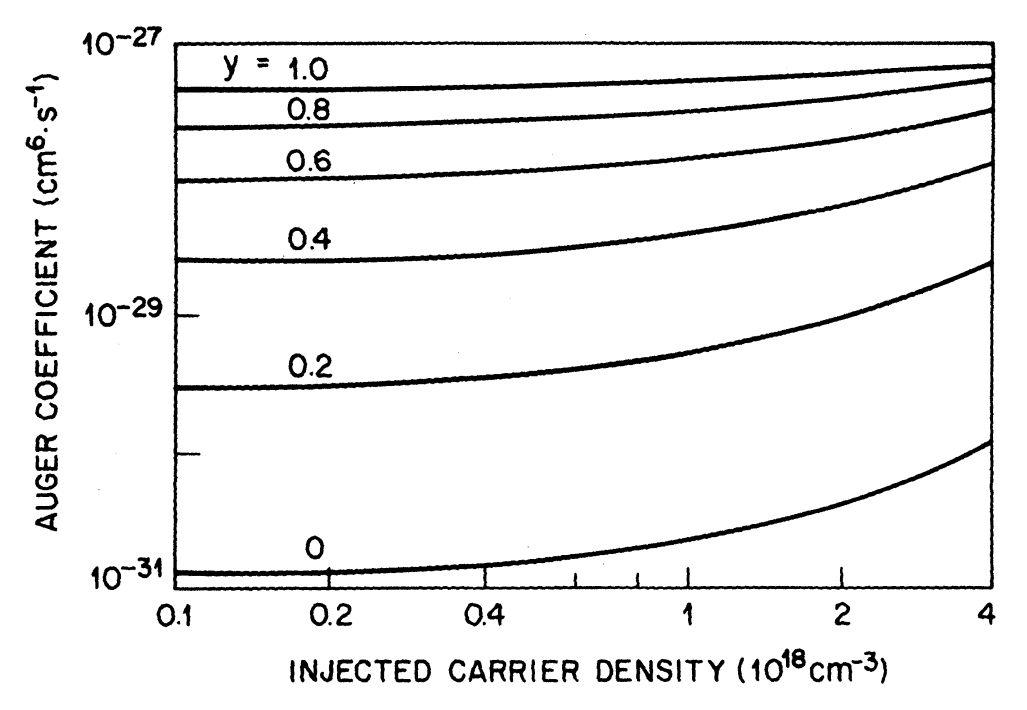Figure 3-16.  Calculated Auger coefficient (due to CHHS) as a function of injected carrier concentration in In1-xGaxAsyP1-y ($$x\approx0.45y$$) for several compositions.

## Phonon-Assisted Auger Processes

The temperature dependence and the band-gap dependence of band-to-band Auger processes arise from the conservation laws of energy and momentum that the four "free-particle" states involved must satisfy. These laws, as seen in the Band-to-Band Auger Processes section above, give rise to a minimum kinetic-energy requirement or to a threshold energy $$E_\text{T}$$ for the processes.

In phonon-assisted Auger processes, conservation of momentum is satisfied through phonon participation and hence the four particle states need not satisfy conservation of momentum. This eliminates the requirement of minimum kinetic energy.

Phonon-assisted Auger processes in the direct-gap semiconductors were first discussed by Eagles. Figure 3-17 shows these processes schematically, which are labeled CCCHP, CHHSP, and CHHLP.

CCCHP is similar to the CCCH process of Figure 3-13, except that the Coulomb interaction produces a heavy hole in an intermediate state $$\mathrm{I}$$, which then interacts with a phonon and reaches a final state $$1'$$. Thus the phonon-assisted Auger process is a second-order process.

All phonon interactions in Figure 3-17 are shown to take place in the heavy-hole band because, as will be shown later, heavy holes interact more strongly with phonons compared to electrons and light holes.Figure 3-17.  Three different kinds of phonon-assisted Auger processes shown schematically. As in Figure 3-14, electrons are represented by closed circles and holes by open circles. The wavy line shows the emission or absorption of a phonon of wave vector $$\mathbf{q}$$.

Phonon-assisted CCCH Process

We first consider the phonon-assisted CCCH process. Using the same approximation for the matrix elements as before, the expression for the Auger rate per unit volume is given by Equation (3-3-12) but without the $$\delta$$ function representing the momentum conservation, i.e.,

\tag{3-3-42}\begin{align}R_\text{p}=&\left(\frac{8\pi}{\hbar}\right)\left(\frac{1}{8\pi^3}\right)^4\\&\times\displaystyle\iiiint\text{d}^3\mathbf{k}_1\text{d}^3\mathbf{k}_2\text{d}^3\mathbf{k}_1'\text{d}^3\mathbf{k}_2'P_\text{p}(1,1',2,2')|M_\text{if}|^2\delta(E_\text{i}-E_\text{f})\end{align}

where

$\tag{3-3-43}M_\text{if}=\sum_m\frac{\langle{i}|H_\text{ee}|{m}\rangle\langle{m}|H_\text{ep}|{f}\rangle}{E_i-E_m}$

$\tag{3-3-44}H_\text{ee}=V(\mathbf{r}_1-\mathbf{r}_2)=\frac{q^2}{\epsilon|\mathbf{r}_1-\mathbf{r}_2|}\exp(-\lambda|\mathbf{r}_1-\mathbf{r}_2|)$

$\tag{3-3-45}\langle{i}|H_\text{ep}|f\rangle=M(\mathbf{q})[(N_\mathbf{q}+1/2)\pm1/2]^{1/2}$

$$H_\text{ee}$$ is the Coulomb-interaction Hamiltonian between two electrons as in Equation (3-3-4), and $$H_\text{ep}$$ is the electron-phonon-interaction Hamiltonian.

The upper sign in the $$\pm$$ of Equation (3-3-45) is for emission of a phonon, and the lower sign is for absorption of a phonon.

$$M(\mathbf{q})$$ is the strength of the interaction for the emission or absorption of a phonon of wave vector $$\mathbf{q}$$, and $$N_\mathbf{q}$$ denotes the number of phonons of wave vector $$\mathbf{q}$$.

We now use the approximation

\tag{3-3-46}\begin{align}E_m-E_i&\approx{E}_\text{v}(\mathbf{k}_1+\mathbf{k}_2-\mathbf{k}_2')-E_\text{v}(\mathbf{k}_1')\\&\approx\frac{\hbar^2k_2'^2}{2m_\text{v}}=\frac{\mu}{\mu_\text{c}}E_\text{g}\end{align}

where, as before,

$\mu=\frac{m_\text{c0}}{m_\text{v}}\qquad\mu_\text{c}=\frac{m_\text{c0}}{m_\text{c}}$

and $$m_\text{c0}$$ and $$m_\text{c}$$ denote the conduction-band masses at the band edge and at energy $$E_\text{g}$$ above the band edge.

The matrix element of $$H_\text{ee}$$ between two-electron states is given by $$|\overline{M}_\text{if}|^2$$ in Equation (3-3-11). The quantity $$P_\text{p}(1,1',2,2')$$ in Equation (3-3-42) is given by

\tag{3-3-47}\begin{align}P_\text{p}&=(1+2N_\mathbf{q})P(1,1',2,2')\\&=(1+2N_\mathbf{q})\frac{n^2p}{N_\text{c}^2N_\text{v}}\exp\left(\frac{-E_2'+E_\text{g}}{k_\text{B}T}\right)\end{align}

where the second line is obtained for the nondegenerate case after using $$P(1,1',2,2')$$ from Equation (3-3-16).

The factor $$1+2N_\mathbf{q}$$ in Equation (3-3-47) arises from the matrix elements of $$H_\text{ep}$$, as shown in Equation (3-3-45), after including both emission and absorption processes.

The summation over the intermediate states $$|m\rangle$$ in Equation (3-3-43) reduces to a single term because of the momentum conservation:

$\tag{3-3-48}\mathbf{q}=\mathbf{k}_1'+\mathbf{k}_2'-\mathbf{k}_1-\mathbf{k}_2$

With these simplifications, we get the following expression for the phonon-assisted Auger rate in the nondegenerate approximation:

$\tag{3-3-49}R_\text{p}=\left(\frac{8\pi}{\hbar}\right)\left(\frac{1}{8\pi^3}\right)^4\left(\frac{\mu_\text{c}}{\mu{E_\text{g}}}\right)^2\frac{n^2p}{N_\text{c}^2N_\text{v}}\exp\left(\frac{E_\text{g}}{k_\text{B}T}\right)I$

where

\tag{3-3-50}\begin{align}I=&\displaystyle\iiiint|M(\mathbf{q})|^2(1+2N_\mathbf{q})|\overline{M}_\text{if}(k_2')|^2\\&\times\exp\left(-\frac{\hbar^2k_2'^2}{2m_\text{c}k_\text{B}T}\right)\delta(E_\text{i}-E_\text{f})\text{d}^3\mathbf{k}_1\text{d}^3\mathbf{k}_2\text{d}^3\mathbf{k}_1'\text{d}^3\mathbf{k}_2'\end{align}

The quantities $$|M(\mathbf{q})|^2$$ and $$N_\mathbf{q}$$ depend on the type of phonon-scattering process. We first consider the case of acoustic phonons. Here, the scattering is caused by acoustic waves and is expressed in terms of a deformation potential $$D$$. The corresponding matrix element is

$\tag{3-3-51}|M_\text{AC}(\mathbf{q})|^2=\frac{D^2\hbar\omega_\text{p}}{2c_1}$

where $$c_1$$ is the averaged longitudinal elastic constant and $$\hbar\omega_\text{p}$$ is the phonon energy.

The number of acoustic phonons $$N_\mathbf{q}$$ at a temperature $$T$$ is given by

$\tag{3-3-52}N_\mathbf{q}=[\exp(\hbar\omega_\text{p}/k_\text{B}T)-1]^{-1}$

and for $$k_\text{B}T\gg\hbar\omega_\text{p}$$ becomes $$N_\mathbf{q}=k_\text{B}T/\hbar\omega_\text{p}\gg1$$.

Since $$|M(\mathbf{q})|^2(1+2N_\mathbf{q})\approx{D}^2k_\text{B}T/c_1$$ is independent of $$\mathbf{q}$$, the integral $$I$$ may be simplified to yield

$\tag{3-3-53}I_\text{AC}=\frac{D^2k_\text{B}T}{c_1}\frac{128\pi^5m_\text{c0}\mu^2}{105\hbar^2}\left(\frac{\mu_\text{c}}{\mu}\right)^{7/2}I_1$

where

$I_1=\displaystyle\int\limits_{k_\text{g}}^\infty\text{d}k_2'(k_2'^2-k_\text{g}^2)^{7/2}k_2'^2\exp\left(-\frac{\hbar^2k_2'^2}{2m_\text{c}k_\text{B}T}\right)|\overline{M}_\text{if}(k_2')|^2$

and $$k_\text{g}^2=2m_\text{c}E_\text{g}/\hbar^2$$.

In deriving the above, we have neglected the contribution of phonon energy in $$E_\text{f}-E_\text{i}$$.

Several other phonon-scattering mechanisms have been discussed; these include piezoelectric scattering, nonpolar-optical-deformation-potential scattering, and polar optical phonon scattering. These scattering mechanisms are described by different electron-phonon-interaction matrix elements and lead to different values of $$I$$ in Equation (3-3-49) for the Auger rate. In the results presented below, all of these mechanisms were included.

We have discussed the case when phonon scattering takes place in the heavy-hole band. When phonon scattering occurs in the conduction band, the quantity $$E_m-E_\text{i}\approx{E}_\text{g}$$ in Equation (3-3-46).

Thus the Auger rate for conduction-band phonon scattering is smaller by a factor $$\sim|\mu/\mu_\text{c}|^2$$. For 1.3-μm InGaAsP, this factor is $$\sim20$$.

The calculations of CHHSP or CHHLP phonon-assisted Auger processes are discussed in detail in some literatures.

Figure 3-18 shows the calculated phonon-assisted Auger lifetime $$\tau_\text{A}=n/R_\text{p}$$ under low injection ($$n=10^{15}\text{ cm}^{-3}$$) for n-type and p-type InGaAsP with a majority carrier concentration of $$10^{18}\text{ cm}^{-3}$$. The calculation was carried out using the Kane band model and the electron-phonon-interaction parameters of GaAs.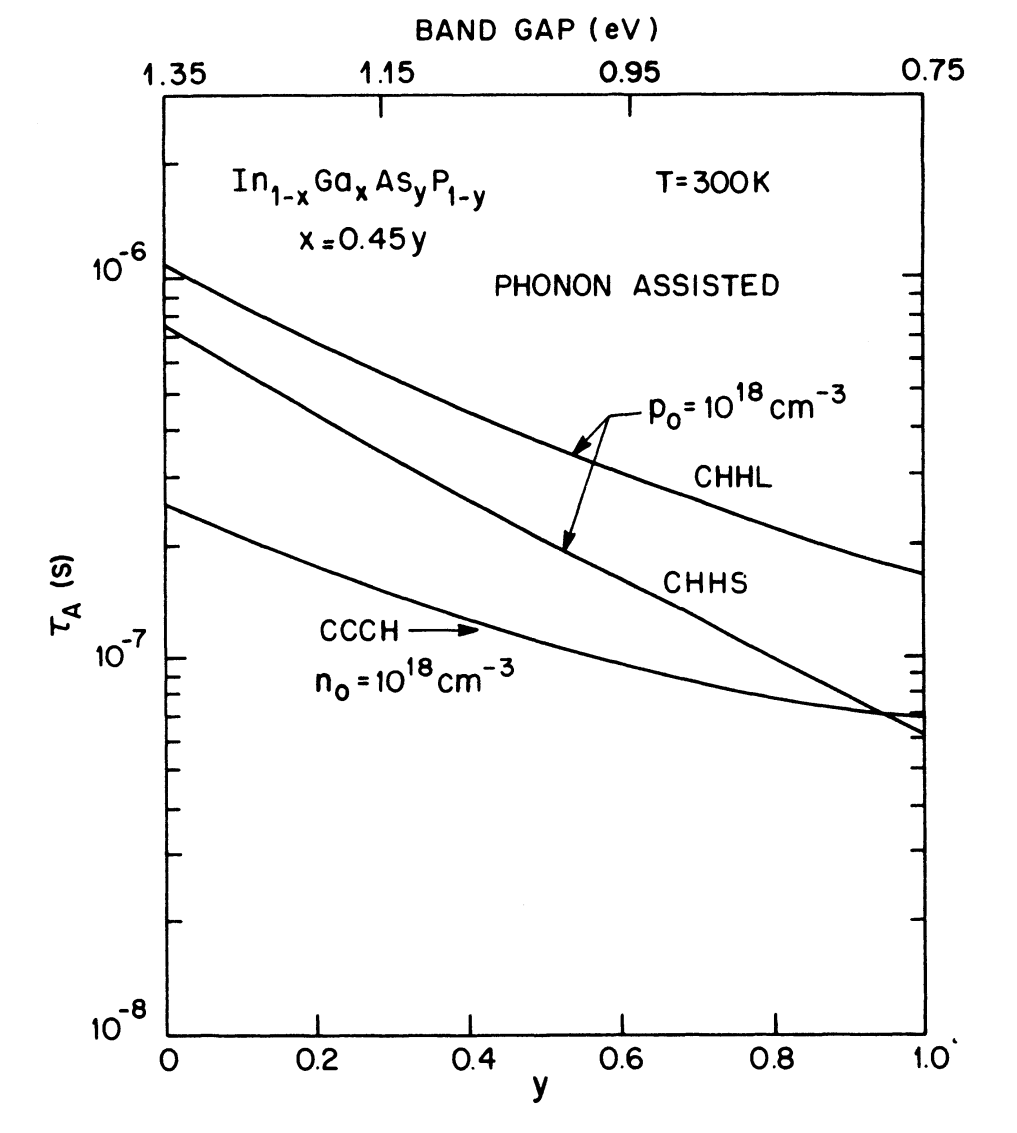Figure 3-18.  Calculated phonon-assisted Auger lifetime for n-type and p-type InGaAsP as a function of the composition (bottom scale) or band gap (top scale). The majority carrier concentration in each case is $$1\times10^{18}\text{ cm}^{-3}$$. Compare with Figure 3-14 drawn for the direct (unassisted) band-to-band Auger process.

Figure 3-18 shows the variation of $$\tau_\text{A}$$ with the band gap of In1-xGaxAsyP1-y lattice-matched to InP ($$x=0.45y$$ and different values of $$y$$ correspond to different band gaps).

CCCH dominates for n-type material, while both CHHL and CHHS are considered for the p-type material.

Figure 3-18 should be compared with Figure 3-14. A direct comparison shows that the dependence of the Auger rate on the band gap is considerably weaker for phonon-assisted processes than for direct band-to-band processes. Further, in both cases the Auger lifetime is shorter for the n-type material when CCCH is dominant.

Figure 3-19 shows the relative contributions of phonon-assisted and band-to-band Auger processes together with the total Auger lifetime for p-type InGaAsP.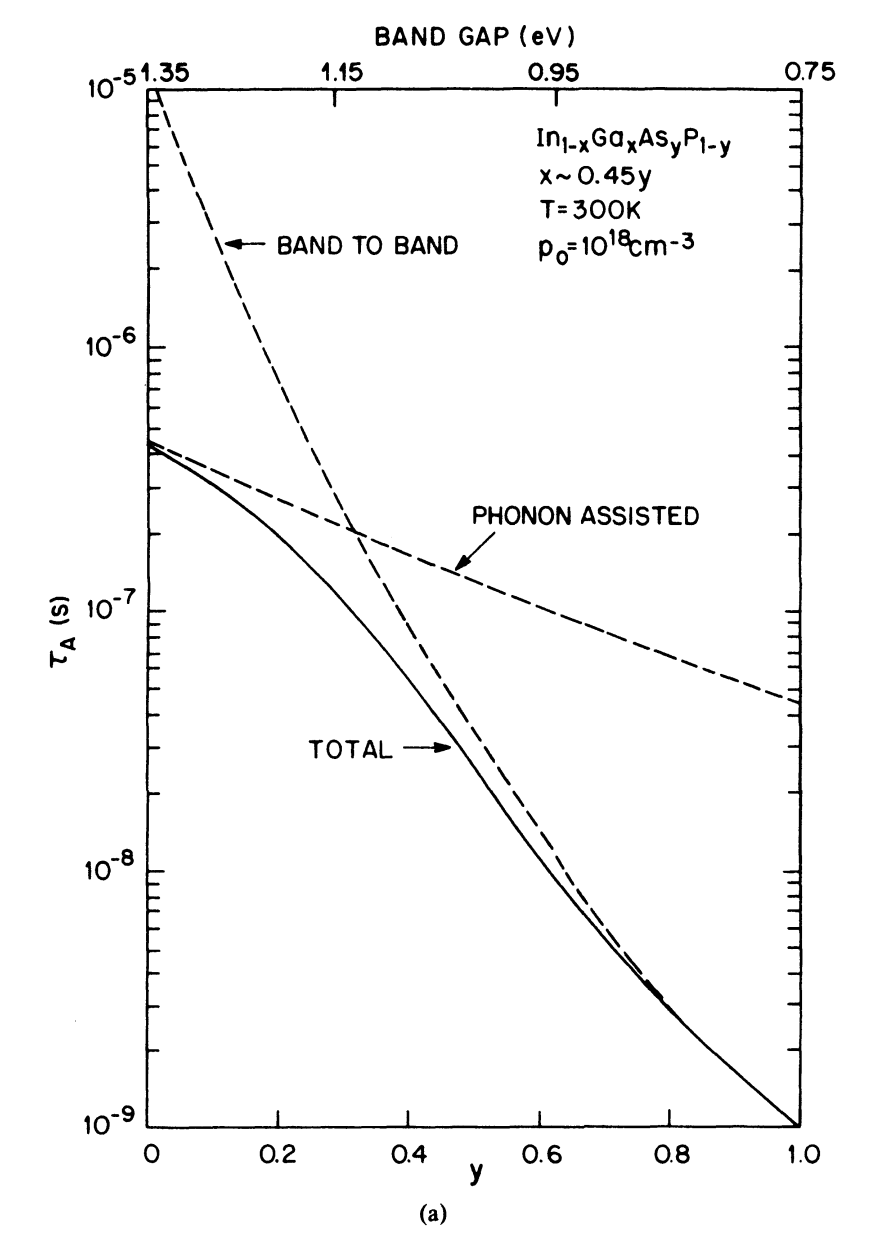Figure 3-19.  Comparison of band-to-band and phonon-assisted Auger processes in InGaAsP.Figure 3-19 (b).  Auger lifetime as a function of temperature for $$E_\text{g}=0.96\text{ eV}$$.

Figure 3-19(a) shows the variation of $$\tau_\text{A}$$ with the band gap at a constant temperature $$T=300\text{ K}$$. We note that when $$E_\text{g}\lt1.14\text{ eV}$$, the band-to-band Auger process dominates.

Figure 3-19(b) shows the variation of $$\tau_\text{A}$$ with temperature at a band gap of 0.96 eV ($$y=0.6$$) corresponding to the wavelength $$\lambda=1.3$$ μm. The band-to-band Auger process dominates for temperatures exceeding $$T=160\text{ K}$$.

Although these results are strongly influenced by the choice of material parameters and band-structure parameters, the qualitative features are expected to be valid.

Haug has calculated the phonon-assisted and band-to-band Auger rates for 1.3-μm InGaAsP using the Chelikowsky and Cohen model of the band structure. He finds that phonon-assisted CCCH and band-to-band CHHS are comparable in magnitude.

It is important to point out that the numerical values calculated for the various Auger processes are strongly influenced (by as much as one order of magnitude) not only by the magnitude of the matrix elements but also by the band structure model used to calculate the effective masses far from the band edge.

Consequently the experimental results provide the only guide for assessing the relative importance of the various Auger processes. These are discussed in the next tutorial.

## Defect and Surface Recombination

Recombination at Defects

Defects in the active region of an injection laser can be formed in several different ways. In many cases they are grown-in during epitaxy. They can also occur, multiply, or propagate during a stress-aging test.

Defects can propagate along a specific crystal axis in a strained lattice. The well-known dark-line defect (dark region of linear aspect) is generally believed to be responsible for the high degradation rates (short life span) of early AlGaAs lasers.

Defects produce a continuum of states in a localized region. Electrons or holes that are within a diffusion length from the edge of the defect may recombine nonradiatively via the continuum of states.

The rate of recombination at a defect or trap is usually written as

$\tag{3-3-54}R_\text{d}=A_\text{nr}n$

where

$\tag{3-3-55}A_\text{nr}=\sigma{v}N_\text{t}$

$$\sigma$$ is the capture cross section of the trap, $$N_\text{t}$$ is the density of traps, and $$v$$ is the velocity of the electrons or holes.

The quantity $$A_\text{nr}$$ is the inverse of the nonradiative lifetime in the presence of a trap, introduced in the gain and stimulated emission in semiconductor lasers tutorial to account for nonradiative recombinations.

Surface Recombination

In an injection laser the cleaved facets are surfaces exposed to the ambient. In addition, in many index-guided laser structures, the edges of the active region can be in contact with curved surfaces that may not form a perfect lattice.

In general, a surface is a strong perturbation of the crystal lattice, creating many dangling bonds that can absorb impurities from the ambient. Hence a high concentration of defects can occur on these surfaces, and the defects can act as centers of nonradiative recombination.

Such localized nonradiative centers, in addition to increasing the threshold current, can cause other performance problems in lasers (e.g., sustained oscillations).

Another possible source for surface recombination is the heterostructure interface between the active and cladding layers.

The recombination rate of carriers at the surface is expressed in terms of a surface recombination velocity. If $$A$$ is the surface area and $$n_\text{th}$$ is the threshold carrier density, then the surface-recombination rate is given by

$\tag{3-3-56}R_\text{s}=SAn_\text{th}$

where $$S$$ is the surface recombination velocity.

For an InP surface exposed to air $$S$$ is about two orders of magnitude smaller than that for GaAs.

For the modeling of semiconductor lasers, it is customary to lump defect and surface recombination and introduce a single parameter $$A_\text{nr}$$, as done in Equation (2-4-9) [refer to the gain and stimulated emission in semiconductor lasers tutorial].

The next tutorial shows the experimental results of radiative and Auger recombination coefficients in semiconductor lasers.